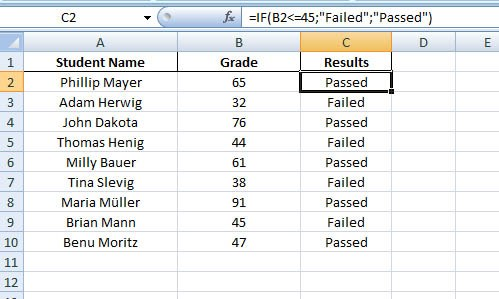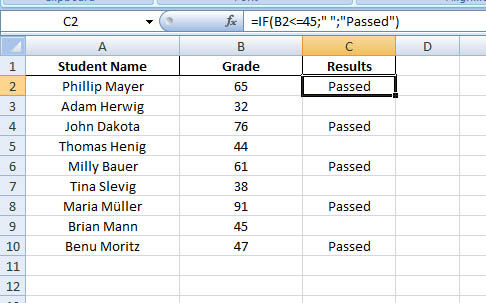Page 1 of 1

### How to use 'IF function' in Excel

Posted: Sun Dec 25, 2016 9:41 pm
What is IF function in Excel?

IF function

- is a logical function

- that checks the condition you put and returns you one value if the condition is TRUE, else the other value if the condition is FALSE

So the syntax for IF function is:

=IF(condition, value_if_true, value_if_false)

It simply says, if the condition is met, then take the value given for value_if_true, but if the condition is not met, then take the value given for value_if_false. That's it.

Let's check this easy table: Students GradeSo here you have a table with students name and grades. In Column C you want to show the results who passed the exam and who failed. In cell C2 enter the if statement

=IF(B2<=45;"Failed";"Passed")

It says, if the student grade is equal to or less than 45, then put Failed in cell C2, otherwise put Passed.

Now copy the formula down the Column C.

However, if you would like to show the results only when a student is passed, and for the failed ones you want to keep the cells blank, then type the following in cell C2:

=IF(B2<=45;"";"Passed")

Copy it down the Column C.

To show the cells blank use double quotation marks ("") without space in-between.So this is your first lesson with the if function*Recommended:

Read wonderful articles (English & German) on Science & Tech, Environment, Health and many other topics only on BlogArena.